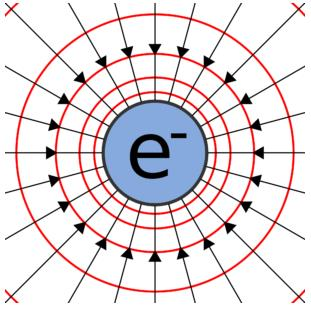Courses

# Basic properties Physics Notes | EduRev

## Physics : Basic properties Physics Notes | EduRev

The document Basic properties Physics Notes | EduRev is a part of the Physics Course Basic Physics for IIT JAM.
All you need of Physics at this link: Physics

Electric Charge Definition

Electric Charge is nothing but the amount of energy or electrons that pass from one body to another by different modes like conduction, induction or other specific methods. This is a basic electric charge definition. There are two types of electric charges. They are positive charges and negative charges.
Charges are present in almost every type of body. All those bodies having no charges are the neutrally charged ones. We denote a charge y the symbol ‘q’ and its standard unit is Coulomb. Mathematically, we can say that a charge is the number of electrons multiplied by the charge on 1 electron. Symbolically, it is

Q = ne

where q is a charge, n is a number of electrons and e is a charge on 1 electron (1.6 × 10-19C). The two very basic natures of electric charges are

• Like charges repel each other.
• Unlike charges attract each other.

This means that while protons repel protons, they attract electrons. The nature of charges is responsible for the forces acting on them and coordinating the direction of the flow of them. The charge on electron and proton is the same in magnitude which is 1.6 × 10-19 C. The difference is only the sign that we use to denote them, + and -.

## Basic Properties of Electric Charge

There are certain other basic properties that an electric charge follows from the electric charge definition. They are

• Charges are additive in nature
• A charge is a conserved quantity
• Quantization of charge

Let us now look at these properties in greater detail.

This means that they behave like scalars and we can add them directly. As an example, let us consider a system which consists of two charges namely q1 and q2.  The total charge of the system will be the algebraic sum of q1 and q2 i.e.q1 + q2. The same thing holds for a number of charges in a system. Let’s say a system contains q1,q2,q3,q………. qn, then the net charge of the entire system will be = q1 + q2 + q3 + q4 + ……. + qnCharge is a Conserved Quantity

This implies that charge can neither be created nor be destroyed but can be transferred from one body to another by certain methods like conduction and induction. Does this remind you of the law of conservation of mass? As charging involves rubbing two bodies, it is actually a transfer of electrons from one body to another.
For example, if 5 C is the total charge of the system, then we can redistribute it as  1C, 2C, and 2C or in any other possible permutation. For example, sometimes a neutrino decays to give one electron and one proton by default in nature. The net charge of the system will be zero as electrons and protons are of the same magnitude and opposite signs.

Quantization of Charge

This signifies the fact that charge is a quantized quantity and we can express it as integral multiples of the basic unit of charge (e – charge on one electron). Suppose charge on a body is q, then we can write it as

q = ne

where n is an integer and not fraction or irrational number, like ‘n’ can be any positive or negative integer like 1, 2, 3, -5, etc. The basic unit of charge is the charge that an electron or proton carries. By convention, we take charge of the electron as negative and denote it as “-e” and charge on a proton is simply “e”.
English experimentalist Faraday was the first to propose the quantization of charge principle. He did this when he put forward his experimental laws of electrolysis. Millikan in 1912, finally demonstrated and proved this principle.
1 A Coulomb of charge contains around 6 × 1018 electrons. Particles don’t have a high magnitude of charge and we use micro coulombs or milli coulombs in order to express charge of a particle.

• 1 μC = 10-6 C
• 1 mC = 10-3 C

We can use the principle of quantization to calculate the total amount of charge present in a body and also to calculate a number of electrons or protons in a body. Suppose a system has n1 number of electrons and n2 number of protons, then the total amount of charge will be n2e – n1e.

## Solved Example For You

Question: The charges of a system are +3 C, + 2 C, + 5 C and -4 C respectively. What would be the net charge of the system?
Solution: We know that the net charge of a system is the algebraic sum of individual charges. Let the total charge of the system be “Q”. Then,
Q = 3 C + 2 C + 5C – 4C
= 6 C

Offer running on EduRev: Apply code STAYHOME200 to get INR 200 off on our premium plan EduRev Infinity!

,

,

,

,

,

,

,

,

,

,

,

,

,

,

,

,

,

,

,

,

,

;